## The tribulations of an interval

### NNT’s confidence interval

The number needed to treat (NNT) is an impact measure that tells us in a simple way about the effectiveness of an intervention or its side effects. If the treatment tries to avoid unpleasant events, the NNT will show us an appreciation of the patients that we have to submit to treatment to avoid one of these events. In this case we talk about NNTB, the number to deal with to benefit.

In other cases, the intervention may produce adverse effects. Then we will talk about the NNTH or number to try to harm one (produce an unpleasant event).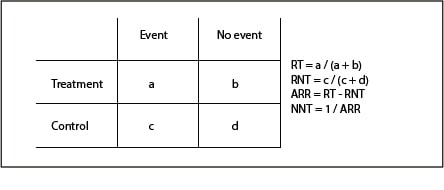The calculation of the NNT is simple when we have a contingency table like the one we see in the first table. It is usually calculated as the inverse of the absolute risk reduction (1 / ARR) and is given as a point estimate value. The problem is that this ignores the probabilistic nature of the NNT, so the most correct would be to specify its 95% confidence interval (95CI), as we do with the rest of the measures.

We already know that the 95CI of any measure responds to the following formula:

95CI (X) = X ± (1.96 x SE (X)), where SE is the standard error.

Thus the lower and upper limits of the interval would be the following:

X – 1.96 SE (X), X + 1.96 SE (X)

#### The tribulations of NNT’s confidence interval

And here we have a problem with the NNT’s 95CI. This interval cannot be calculated directly because NNT does not have a normal distribution. Therefore, some tricks have been invented to calculate it, such us to calculate the 95CI of the ARR and use its limits to calculate the NNT’s, as follows:

95CI (ARR) = ARR – 1,96(SE(ARR)) , ARR + 1,96(SE(ARR))

CI(NNT) = 1 / upper limit of the 95CI (ARR), 1 / lower limit of the 95CI (ARR) (we use the upper limit of the ARR to calculate the lower limit of the NNT, and vice versa, because being the treatment beneficial, risk reduction would in fact be a negative value [RT – RNT], although we usually speak of it in absolute value).

We just need to know how to calculate the RAR’s SE, which turns out to be done with a slightly unfriendly formula that I put to you just in case anyone is curious to see it: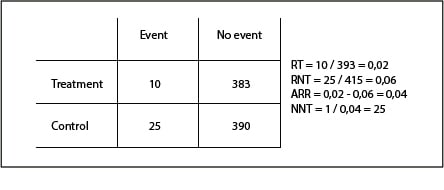In the second table you can see a numerical example to calculate the NNT and its interval. You see that the NNT = 25, with an 95CI of 15 to 71. Look at the asymmetry of the interval since, as we have said, does not follow a normal distribution. In addition, far from the fixed value of 25, the interval values say that in the best case we will have to treat 15 patients to avoid an adverse effect, but in the worst case this value can rise to 71.

To all the above difficulty for its calculation, another added difficulty arises when the ARR’s 95CI includes zero. In general, the lower the effect of the treatment (the lower the ARR) the higher the NNT (it will be necessary to treat more to avoid an unpleasant event), so in the extreme value of the effect is zero, the NNT’s value will be infinite (an infinite number of patients would have to be treated to avoid an unpleasant event).

So it is easy to imagine that if the 95CI of the ARR includes zero, the 95CI of the NNT will include infinity. It will be a discontinuous interval with a negative value limit and a positive one, which can pose problems for its interpretation.

For example, suppose we have a trial in which we calculated an ARR of 0.01 with a 95CI of -0.01 to 0.03. With the absolute value we have no problem, the NNT is 100 but, what about with the interval? For it would go from -100 to 33, going through infinity (actually, from minus infinity to -100 and from 33 to infinity).

How do we interpret a negative NNT? In this case, as we have already said, we are dealing with an NNTB, so its negative value can be interpreted as a positive value of its alter ego, the NNTH. In our example, -100 would mean that we will have an adverse effect for every 100 treated. In short, our interval would tell us that we could produce one event for every 100 treated, in the worst case, or avoid one for every 33 treated, in the best. This ensures that the interval is continuous and includes the point estimate, but it will have little application as a practical measure. Basically, it may make little sense to calculate the NNT when the ARR is not significant (its 95CI includes zero).

#### We’re leaving…

At this point, the head begins to smoke us out, so let’s go ending today. Needless to say, everything I have explained about the calculation of the interval can be done clicking with any of the calculators available on the Internet, so we will not have to do any math.

In addition, although the NNT calculation is simple when we have a contingency table, we often have adjusted risk values obtained from regression models. Then, the maths for the calculation of the NNT and its interval gets a little complicated. But that is another story…

That is bootstrapping. It’s an idea impossible to perform, in addition to a swearword, of course.

The name is related to the straps that boots have on their top, especially those cowboy’s boots we see in the movies. Bootstrapping is a term that apparently refers to the action of elevated oneself from the ground by simultaneously pulling the straps of both boots. As I said, it’s an impossible task because of the Newton’s third law, the famous action-reaction law.

Bootstrapping is a resampling technique that is used in statistics with increasing frequency thanks to the power of today’s computers, which allow calculations that could previously be inconceivable. Perhaps his name has to do with its character of impossible task because bootstrapping is used to make possible some tasks that might seem impossible when the size of our sample is very small or when the distributions are highly skewed, as obtaining confidence intervals or perform statistical significance tests or any other statistics in which we are interested.

As you recall from when we calculate the mean’s confidence interval, in theory we can do the experiment of obtaining multiple samples from a population to calculate the mean of each sample and represent the distribution of those means obtained from multiple samples. This is called the sampling distribution, whose mean is the estimator of the parameter in the population and whose standard deviation is called the standard error of the statistic that, in turn, will allow us to calculate the confidence interval we want. Thus, extraction of repeated samples from the population allows us to make descriptions and statistical inferences.

Well, bootstrapping is similar, but with one key difference: the successive samples are taken from our sample and not from the population from which it comes. The procedure follows a series of repetitive steps.

First, we draw a sample from the original sample. This sample must be collected using sampling with replacement, so some items may not be selected and others may be selected more than once in each sampling. It is logical, if we have a sample of 10 elements and extract 10 items without replacement, the sample obtained is equal to the original, so that we won’t get anything new.

From this new sample we get the desired statistic and use it as an estimator of the value in the population. As this estimate would be inaccurate, we repeat the previous two steps a number of times, thus obtaining a high number of estimates.

We’re almost there. With all these estimators we construct its distribution which we call bootstrap distribution, which represents an approximation of the true distribution of the statistic in the population. Obviously, this requires that the original sample from which we start is representative of the population. The more different from the population, the less reliable the approximation of the distribution we’ve calculated.

Finally, using the bootstrap distribution we can calculate its central value (the point estimator) and its confidence interval in a similar way as we did for calculating the mean’s confidence interval with the sampling distribution.

As you can see, a very nimble procedure that nobody would dare to implement without the help of a statistical program and a good computer. Let’s see a practical example for better understanding.

Let’s suppose for a moment that we want to know what the intake of alcohol in a certain group of people is. We collected 20 individuals and calculate their weekly alcohol consumption in grams, with the following results: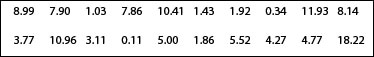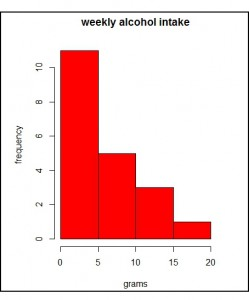You can see the data plotted in the first histogram. As you see, the distribution is asymmetric with a positive bias (to the right). We have a dominant group of teetotalers and scarce drinkers with a tail that represents to those who are taking increasingly higher intakes, which are becoming less frequent. This type of distribution is very common in biology.

In this case the mean would not be a good measure of central tendency, so we prefer to calculate the median. To do this, we can sort the values from lowest to highest and make the average of those in the tenth and eleventh places. I’ve bothered to do it and I know that the median equals (4.77 + 5) / 2 = 4.88.

But now, I’m interested in knowing the value of the median in the population from which the sample comes. The problem is that with such a small and biased sample I cannot apply the usual procedures and I’m not able to collect more individuals from the population to perform the calculation using them. Here’s when bootstrapping comes in handy.

So I obta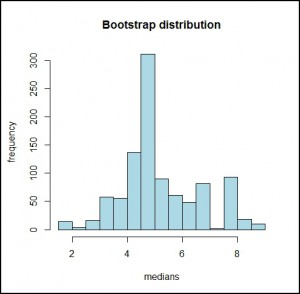in 1000 samples with replacement from my original sample and calculate the medians of the 1000 samples. The bootstrap distribution of these 1000 medians is represented in the second histogram. As can be seen, it looks like a normal distribution whose mean is 4.88 and whose standard deviation is 1.43.

Well, we can now calculate our confidence interval for the population estimate. We can do this in two ways. First, calculating the margins which cover 95% of the bootstrap distribution (calculating the 2.5th and the 97.5th percentiles), as you can see represented in the third graph. I used the program R, but it can be done manually using formulas to calculate percentiles (although it’s not highly recommended, as there are 1000 medians to deal with). So, I get a mean of 4.88 with a 95% confidence interval from 2.51 to 7.9.

The other way is using the central limit theorem that we cannot use with the sampling distribution but can use wi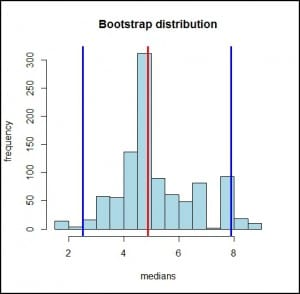th the bootstrap distribution. We know that the 95% confidence interval is equal to the median plus and minus 1.96 times the standard error (which is the standard deviation of the bootstrap distribution). Then:

95% CI = 4,88 ± 1,96 x 1,43 = 2,08 a 7,68.

As you see, it looks pretty similar to that obtained with the percentile’s approximation.

And here we leave the matter for today, before any head overheats in excess. To encourage you a little, all this crap can be avoided resorting to a software like R, which calculates the interval and makes the bootstrapping if necessary, with such a simple command as ci.median() from the asbio library.

This is all for today. Just saying that bootstrapping is perhaps the most famous of the resampling techniques, but it’s not alone. There’re more, some with peculiar names such as jackknife, randomization and validation test or cross-validation test. But that’s another story…

## With very little we fine-tune a lot

We all like to know what will happen in the future. So we try to invent things that help us to know what will happen, what will be the result of a certain thing. A clear example is the political elections or surveys to ask people on an issue of interest. So the polls have been invented to try to anticipate the outcome of a survey before happening. Many people do not trust polls, but as discussed below, they are a very useful tool: they allow us to make estimates with relatively little effort.

Consider, for example, that we do a Swiss-style referendum to ask people if they want to reduce their workday. Some of you will tell me that this is a waste of time, since a survey like that in Spain would have a very predictable result, but you never know. In Switzerland they asked and people preferred to continue working longer.

If we wanted to know for sure what will be the outcome of the voting, we’d have to ask everyone what their vote will be, which is impractical to carry out. So we do a poll: we select a sample of a given size and asked them. We obtain an estimate of the final result, with an accuracy that is determined by the confidence interval of the calculated estimator.

But, will the sample have to be very large?. Well, not too much, if it’s well chosen. Let’s see it.

Every time we do the poll we obtain a value of the p proportion that will vote, for instance, yes to the proposal we asked for. If we repeated the poll many times, we get a set of values ​​close to each other and probably close to the actual value of the population that we cannot access. Well, these values ​​(result of the different repeated polls) follow a normal distribution, so we know that 95% of the values ​​would be between the value of the proportion in the population plus or minus two times the standard deviation (actually, 1.96 times the standard deviation). This standard deviation is called the standard error, and is the measure that allows us to calculate the margin of error of the estimation by its confidence interval:

95% confidence interval (95 CI) = estimated proportion ± 1.96 x standard error

Actually, this is a simplified equation. If we start from a finite sample (n) obtained from a population (N), the standard error should be multiplied by a correction factor, so that the formula is as follows:

If you think about it for a moment, when the population is large the ratio n / N tends to zero, so that the result of the correction factor tends to one. This is the reason why the sample not needs to be excessively large, and why the same sample size can serve to estimate the results of an election in a little town or in the entire nation.

Therefore, the estimation accuracy is more related to the standard error. What would be the standard error in our example?. As the result is a proportion, we know it follows a binomial distribution, so the standard error is equal to , where p is the proportion obtained and n the sample size.

The imprecision (the amplitude of the confidence interval) will be greater the larger the standard error. Therefore, the greater the product p (1-p) or the smaller the sample size, the less accurate will be our estimate and the greater our margin of error.

Anyway, this margin of error is limited. Let’s see why.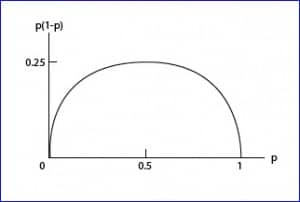We know that p can have values ​​between zero and one. If we examine the figure with the curve of p vs p(1-p), we see that the maximum value of the product is obtained when p = 0.5, with a value of 0.25. As p moves away from 0.5 in either direction, the product will be lesser.

So, for a given value of n, the standard error is maximum when p equals 0.5, using the following equation:

Thus, we can write the formula of the maximum confidence interval:

That is, the maximum margin of error is  . This means that with a sample of 100 people we will have a maximum margin of error of plus or minus 10%, depending on the value of p we have obtained (but a maximum of 10%). Thus we see that with a sample that not need to be very large, we can get a fairly accurate result.

And with that we’re done for today. You might ask, after all we have said, why there are polls whose result is different from the definitive result. Well, I can think of two reasons. First, random. We have been able to choose, by chance, a sample that is not centered on the true value of the population (it will happen 5% of the times). Second, the sample may not be representative of the general population. And this is a key factor, because if the sampling technique is incorrect, the results of the survey will be unreliable. But that’s another story…

## The error of confidence

Our life is full of uncertainty. There’re many times that we want to know information that is out of our reach, and then we have to be happy with approximations. The problem is that approximations are subject to error, so we can never be completely sure that our estimates are true. But do we can measure our degree of uncertainty.

This is one of the things statistics is responsible for, quantifying uncertainty. For example, let’s suppose we want to know the mean cholesterol levels of adults between 18 and 65 years from the city where I live in. If we want the exact number we have to call them all, convince them to be analyzed (most of them are healthy and won’t want to know anything about analysis) and make the determination to every one of them to calculate the average we want to know.

The problem is that I live in a big city, with about five million people, so it’s impossible from a practical point of view to determine serum cholesterol to all adults from the age range that we are interested in. What can we do?. We can select a more affordable sample, calculate the mean cholesterol of their components and then estimate what is the average value in the entire population.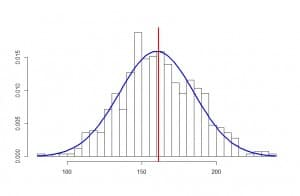So, I randomly pick out 500 individuals and determine their cholesterol levels, in milligrams per deciliter, getting a mean value of 165, a standard deviation of 25, and an apparently normal distribution, as it’s showed in the graph attached.

Logically, as the sample is large enough, the average value of the population will probably be close to that of 165 obtained from the sample, but it’s also very unlikely to be exactly that. How can we know the value of the population? The answer is that we cannot know the exact value, but we can know what the approximate value is. In other words, we can calculate a range within which the value of my unaffordable population is, always with a certain level of confidence (or uncertainty) that can be settled by us.

Let’s consider for a moment what would happen if we repeat the experiment many times. We would get a slightly different value every time, but all of them should be similar and close to the actual value of the population. If we repeat the experiment a hundred times and get a hundred mean values, these values will follow a normal distribution with a specific mean and standard deviation.

Now, we know that, in a normal distribution, about 95% of the sample is located in the interval enclosed by the mean plus minus two standard deviations. In the case of the distribution of means of our experiments, the standard deviation of the means distribution is called the standard error, but its meaning is the same of any standard deviation: the range between the mean plus minus two standard errors contains 95% of the means of the distribution. This implies, roughly, that the actual mean of our population will be included in that interval 95% of the times, and that we don’t need to repeat the experiment a hundred times, it’s enough to compute the interval as the obtained mean plus minus two standard errors. And how can we get the mean’s standard error? Very simple, using the following expression:

standard error = standard deviation / square root of sample size

In our example, the standard error equals 1.12, which means that the mean value of cholesterol in our population is within the range 165 – 2.24 to 165 + 2.24 or, what is the same, 162.76 to 167.24, always with a probability of error of 5% (a level of confidence of 95%).

We have thus calculated the 95% confidence interval of our mean, which allow us to estimate the values between which the true population mean is. All confidence intervals are calculated similarly, varying in each case how to calculate the standard error, which will be different depending on whether we are dealing with a mean, a proportion, a relative risk, etc.

To finish this post I have to tell you that the way we have done this calculation is an approximation. When we know the standard deviation in the population we can use a standard normal distribution to calculate the confidence interval. If we don’t know it, which is the usual situation, and the sample is large enough, we can make an approximation using a normal distribution committing little error. But if the sample is small the distribution of our means of repetitive experiments won’t follow a normal distribution, but a Student’s t distribution, so we should use this distribution to calculate the interval. But that’s another story…

## Life is not rosy

We, the so-called human beings, tend to be too categorical. We love to see things in black and white, when the reality is that life is neither black nor white, but manifest itself in a wide range of grays. Some people think that life is rosy or that the color lies in the eye of the beholder, but do not believe it: life if gray colored.

And, sometimes, this tendency to be too categorical leads us to very different conclusions about a particular topic depending on the white or black eye that the beholder has. So, it’s not uncommon to observe opposing views on certain topics.

And the same can happen in medicine. When there’s a new treatment and it starts running papers about its efficacy or toxicity, it’s not uncommon to find similar studies in which the authors come to very different conclusions. In many times this is due to the effort we do to see things in black or white, drawing categorical conclusions based on parameters like statistical significance, the value of p. Actually, data in many cases don’t say so different things, but we have to look at the range of grays provided to us by confidence intervals.

As I imagine you do not understand quite well what the heck I’m talking about, I’ll try to explain myself better and to give an example.

You know that we can never ever prove the null hypothesis. We can only be able or unable to reject it (in this last case we assume that it’s true, but with a probability of error). This is why when we study the effect of an intervention we state the null hypothesis that the effect does not exist and we design the trial to give us the information about whether or not we can reject it. In case of rejecting it, we assume the alternative hypothesis that says that the effect of the intervention exists. Again, always with a probability of error; this is the p-value or statistical significance.

In short, if we reject the null hypothesis we assume that the intervention has an effect and if we cannot reject it we assume the effect doesn’t exist. Do you realize?: black or white. This so simplistic interpretation doesn’t consider all the grays related to important factors such us clinical relevance, the precision of the estimation or the power of the study.

In a clinical trial it is usual to provide the difference found between the intervention and control groups. This is a punctual estimation but, as we have performed the trial with a sample from a population, the right thing is to complement the estimate with a confidence interval that provides the range of values that includes the true value in the inaccessible population with a certain probability or confidence. By convention, confidence is usually set at 95%.

This 95% value is usually chosen because we also often use a statistical significance level of 5%, but we must not forget that these are arbitrary values. The great quality of confidence intervals, opposite to p-values, is that no dichotomous conclusions (the kind of white or black) can be drawn.

A confidence interval is not statistically significant when it intersects the line of no effect, which is 1 for relative risks and odds ratios and 0 for absolute risks and mean differences. If you just look at the p-value you can only conclude if the interval reached or not statistical significance, coming up sometimes to very different conclusions with very similar intervals.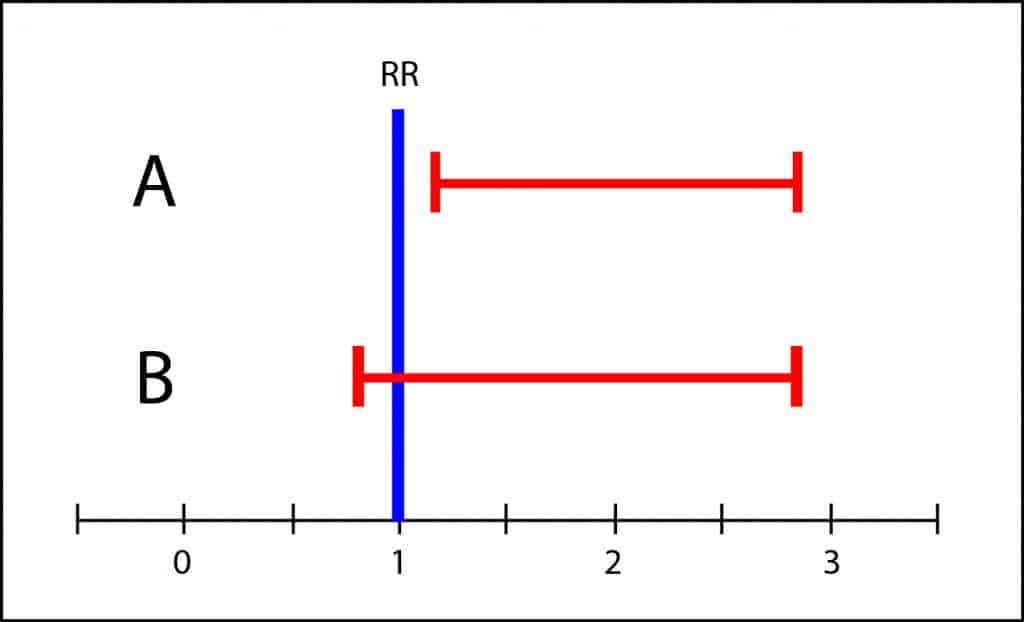Let’s see an example. The graph shows the confidence intervals of two studies on the cardiovascular adverse effects of a new treatment. Notice that both intervals are very similar, but trial A’s is statistically significant while B’s is not. If the authors were those of black or white, authors of trial A would say that treatment has cardiovascular toxicity, whereas those of B would say that there’s not statistically significant difference between intervention and control groups in relation to cardiovascular toxicity.

However, the interval of B covers from slightly less than 1 to about 3. This means that the population’s value may be any value in the interval. It could be 1, but it could be also 3, so it’s not impossible that toxicity in the intervention group could be three times greater than in the control group. If the side effects were serious, it wouldn’t be appropriate to recommend the treatment until more conclusive studies with more precise intervals were available. This is what I mean by the scale of grays. It is unwise to draw black or white conclusions when there’s overlapping of confidence intervals.

So better follow my advice. Pay less attention to p-values and always seek the information about the possible range of effect provided by confidence intervals.

And that’s all for now. We could talk more about similar situations but when dealing with efficacy studies, or superiority or non-inferiority studies. But that’s another story…

## Even non-significant Ps have a little soul

In any epidemiological study, results and validity are always at risk of two fearsome dangers: random bias and systematic bias.

Systematic bias (or systematics errors) are related to study design defects in any of its phases, so we must be careful to avoid them in order to not to compromise the validity of the results.

Random bias is quite different kettle of fish. It’s inevitable and is due to changes beyond our control which occur during the process of measurement and data collection, so altering the accuracy of our results. But do not despair: we can’t avoid randomness, but we can control (within some limits) and quantify it.

Let’s suppose we have measured differences in oxygen saturation between lower and upper extremities in twenty healthy newborns and we’ve came up with an average result of 2.2%. If we repeat the experiment, even in the same infants, what value will we come up with?. In all probability, any value but 2.2% (although it will seem quite similar if we make the two rounds in the same conditions). That’s the effect of randomness: repetition tends to produce different results, although always close to the true value we want to measure.

Random bias can be reduced by increasing the sample size (with one hundred instead of twenty children the averages will be more the same if we repeat the experiment), but we’ll never get rid of it completely. To make things worse, we don’t even want to know the mean saturation’s differences in these twenty, but in the overall population from which they are extracted. How can we get out of this maze?. You’ve got it, using confidence intervals.

When we establish the null hypothesis of no difference between measuring saturation on the leg or on the arm and we compare means with the appropriate statistical test, p-values will tell us the probability that the difference found is due to chance. If p<0.05 we’ll assume that the probability it is due to chance is small enough to calmly reject the null hypothesis and embrace the alternative hypothesis: it is not the same to measure oxygen saturation on the leg or on the arm. On the other hand, if p is not significant we won’t able to reject the null hypothesis, insomuch us we’ll always think about what if we would have obtained the p-value with 100 children, or even with 1000. p might have reach statistical significance and we might have rejected H0.

If we calculate the confidence interval of our variable we’ll get the range in which the real value is with a certain probability (typically 95%). The interval will inform us about the accuracy of the study. It will not be the same to come up with oxygen saturation’s difference from to 2 to 2.5% than from 2 to 25% (in this case, we should distrust study results no matter it had a five-zero p value).

And what if p is non-significant?. Can we draw any conclusions from the study?. Well, that depends largely on the importance of what we are measuring, on its clinical relevance. If we consider as clinically significant a saturation difference of 10% and the interval is below this value, clinical importance will be low no matter the significance of p. But the good news is that this reasoning can also be state in the reverse way: non-statistically significant intervals can have a great impact if any of its limits intersect with the area of clinical importance.

Let’s see some examples in the figure above, in which a difference of 5% oxygen saturation has been considered as clinically significant (I apologize to the neonatologists, but the only thing I know about saturation is that it’s measured by a device that now and then is not capable of doing its task and beeps).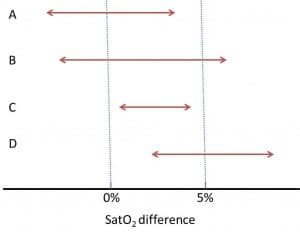Study A is not statistically significant (its confidence interval intersects with the null effect, which is zero in our example) and, also, it doesn’t seem to be clinically important.

Study B is not statistically significant but it may be clinically important, since its upper limits falls into the clinical relevance’s area. If you’d increase the accuracy of the study (increasing sample size), who assures us that the interval could not be narrower and above the null effect line, reaching statistical significance?. In this case the question is not very important because we are measuring a bit nonsense variable, but think about how the situation would change if we were considering a harder variable, as mortality.

Studies C and D reach statistical significance, but only study D’s results are clinically relevant. Study C shows a statistically significant difference, but its clinical relevance and therefore its interest are minimal.

So, you see, there are times that a non-statistically significant p-value can provide information of interest from a clinical point of view, and vice versa. Furthermore, all that we have discussed is important to understand the designs of superiority, equivalence and non-inferiority trials. But that’s another story…